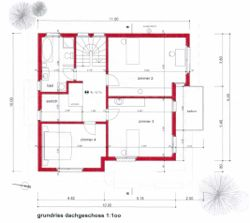deutsch | english | français

Bed and Breakfast Wallis » Visp » Bed and Breakfast / Rooms

# Bed and Breakfast and Rooms in Visp / Oberwallis / Wallis / SwitzerlandOur B&B offers a spacious room on the second floor of a single family house with shared balcony. The room offers a spectacular view on the majestic Mischabel mountain range. TV (132 channels), free access to internet via WiFi and access to a kitchen come as part of the package.

• Free parking
• Walking distance to central station: about 10 minutes
• Double bed (180x200) or 2 twin beds (90x200) on request

## Prices (per person)

 Double Room without Breakfast    Double Room with Breakfast Single Room without Breakfast Single Room with Breakfast from 4 nights     Fr. 40.- Fr. 48.- Fr. 51.- Fr. 59.- 1-3 nights Fr. 45.- Fr. 51.- Fr. 54.- Fr. 62.-

## Availability (Room 2)

 2022 1 2 3 4 5 6 7 8 9 10 11 12 13 14 15 16 17 18 19 20 21 22 23 24 25 26 27 28 29 30 31 June x x x July x x x x x x x x x x x x x x x x x x x x x x x x x x x x x x x August x x x x x x x x x x x x x x x x x x x x x x x x x x x x x x x September x x x x x x x x x x x x x x x x x x x x x x x x x x x x x x x October x x x x x x x x x x x x x x x x x x x x x x x x x x x x x x x November x x x x x x x x x x x x x x x x x x x x x x x x x x x x x x x December x x x x x x x x x x x x x x x x x x x x x x x x x x x x x x x